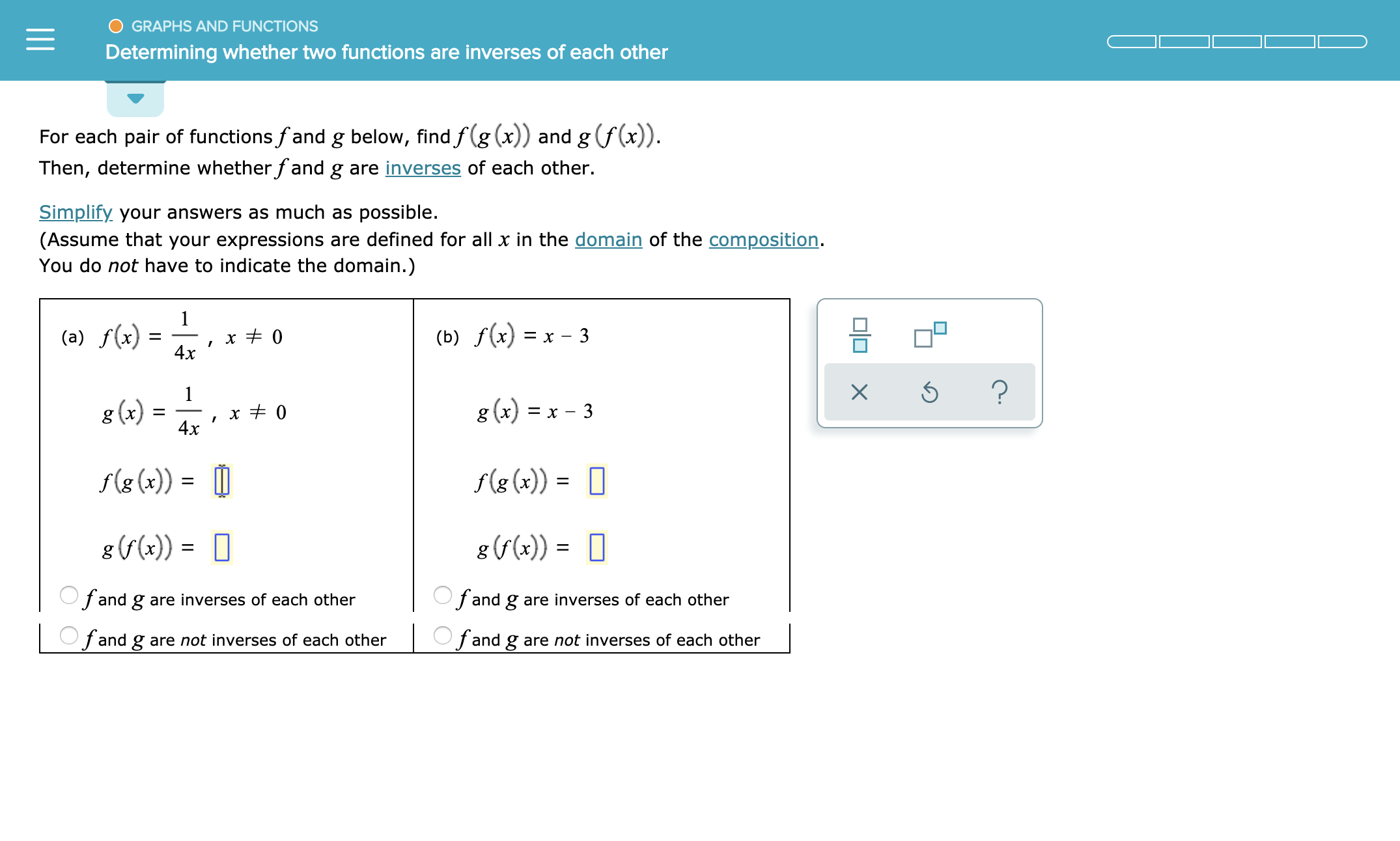GRAPHS AND FUNCTIONSDetermining whether two functions are inverses of each otherFor each pair of functions fand g below, find f(g (x)) and g (f(x))Then, determine whether fand g are inverses of each other.Simplify your answers as much as possible.(Assume that your expressions are defined for all x in the domain of the composition.You do not have to indicate the domain.)1(b) f(x) = x - 3(a) f(x)x 04x?X1g (x)x - 3g (x)x 04xf(g (x)f(g (x)) =g (r(x)) =g (f(x))=Ofand gOfand g are inverses of each otherare inverses of each other|Ofand g are not inverses of each otherfand gare not inverses of each other

Question

see attachmenthelp_outlineImage TranscriptioncloseGRAPHS AND FUNCTIONS Determining whether two functions are inverses of each other For each pair of functions fand g below, find f(g (x)) and g (f(x)) Then, determine whether fand g are inverses of each other. Simplify your answers as much as possible. (Assume that your expressions are defined for all x in the domain of the composition. You do not have to indicate the domain.) 1 (b) f(x) = x - 3 (a) f(x) x 0 4x ? X 1 g (x)x - 3 g (x) x 0 4x f(g (x) f(g (x)) = g (r(x)) = g (f(x))= Ofand g Ofand g are inverses of each other are inverses of each other |Ofand g are not inverses of each other fand gare not inverses of each other fullscreen
Step 1

Step 2

f and g are inverse functions of each other.

Step 3

Want to see the full answer?

See Solution

Want to see this answer and more?

Our solutions are written by experts, many with advanced degrees, and available 24/7

See Solution
Tagged in

Other